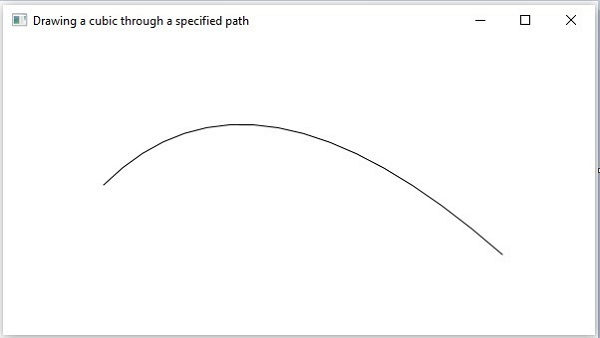# 2D Shapes PathElement Quadratic Curve

The path element quadratic curve is used to draw a quadratic curve to a point in the specified coordinates from the current position.

It is represented by a class named QuadraticCurveTo. This class belongs to the package javafx.scene.shape.

This class has 4 properties of the double datatype namely −

• setX − The x coordinate of the point to which a curve is to be drawn from the current position.

• setY − The y coordinate of the point to which a curve is to be drawn from the current position.

• controlX − The x coordinate of the control point of the curve.

• controlY − The y coordinate of the control point of the curve.

To draw a quadratic curve, you need to pass values to these properties. This can be done either by passing them to the constructor of this class, in the same order, at the time of instantiation, as follows −

```QuadCurveTo quadcurve = new QuadCurveTo(X, Y, controlX, controlY);
```

Or, by using their respective setter methods as shown below −

```setX(value);
setY(value);
setControlX(value);
setControlY(value);
```

## Steps to draw PathElement Quadratic Curve

To draw a quadratic curve to a specified point from the current position in JavaFX, follow the steps given below.

### Step 1: Creating a Class

Create a Java class and inherit the Application class of the package javafx.application and implement the start() method of this class as follows.

```public class ClassName extends Application {
@Override
public void start(Stage primaryStage) throws Exception {
}
}
```

### Step 2: Create the path class object

Create the Path Class Object as shown below.

```//Creating a Path object
Path path = new Path();
```

### Step 3: Create a Path

Create the MoveTo path element and set the XY coordinates to the starting point of the line to the coordinates (100, 150). This can be done by using the methods setX() and setY() of the class MoveTo as shown below.

```//Moving to the starting point
MoveTo moveTo = new MoveTo();
moveTo.setX(100.0f);
moveTo.setY(150.0f);
```

### Step 4: Creating an Object of the Class QuadCurveTo

Create the path element Quadratic Curve by instantiating the class named QuadCurveTo which belongs to the package javafx.scene.shape as follows.

```//Creating an object of the class QuadCurveTo
```

### Step 5:Setting Properties to the Quadratic Curve Element

Specify the coordinates of the point to which a Quadratic Curve is to be drawn from the current position. Then you should set the properties x, y, controlx, controlY and the coordinates of the control point by their setter methods as shown below.

```//Setting properties of the class QuadCurve
```

### Step 6: Adding Elements to the Observable List of the Path Class

Add the path elements MoveTo and QuadraticCurveTo created in the previous steps to the observable list of the Path class as follows −

```//Adding the path elements to Observable list of the Path class
```

### Step 7: Creating a Group Object

Create a group object by instantiating the class named Group, which belongs to the package javafx.scene.

Pass the Line (node) object created in the previous step as a parameter to the constructor of the Group class. This can be done in order to add it to the group as shown below −

```Group root = new Group(line);
```

### Step 8: Creating a Scene Object

Create a Scene by instantiating the class named Scene which belongs to the package javafx.scene. To this class, pass the Group object (root) created in the previous step.

In addition to the root object, you can also pass two double parameters representing height and width of the screen along with the object of the Group class as follows −

```Scene scene = new Scene(group ,600, 300);
```

### Step 9: Setting the Title of the Stage

You can set the title to the stage using the setTitle() method of the Stage class. The primaryStage is a Stage object which is passed to the start method of the scene class as a parameter.

Using the primaryStage object, set the title of the scene as Sample Application as follows.

```primaryStage.setTitle("Sample Application");
```

### Step 10: Adding Scene to the Stage

You can add a Scene object to the stage using the method setScene() of the class named Stage. Add the Scene object prepared in the previous steps using this method as shown below −

```primaryStage.setScene(scene);
```

### Step 11: Displaying the Contents of the Stage

Display the contents of the scene using the method named show() of the Stage class as follows.

```primaryStage.show();
```

### Step 12: Launching the Application

Launch the JavaFX application by calling the static method launch() of the Application class from the main method as follows.

```public static void main(String args[]){
launch(args);
}
```

### Example

Following is a program which draws a quadratic curve from the current point to a specified position using the class named Path of JavaFX. Save this code in a file with the name QuadCurveToExample.java.

```import javafx.application.Application;
import javafx.scene.Group;
import javafx.scene.Scene;
import javafx.stage.Stage;
import javafx.scene.shape.MoveTo;
import javafx.scene.shape.Path;

public class QuadCurveToExample extends Application {
@Override
public void start(Stage stage) {
//Creating an object of the class named Path
Path path = new Path();

//Moving to the starting point
MoveTo moveTo = new MoveTo();
moveTo.setX(100.0);
moveTo.setY(150.0);

//Setting properties of the class QuadCurve

//Adding the path elements to Observable list of the Path class

//Creating a Group object
Group root = new Group(path);

//Creating a scene object
Scene scene = new Scene(root, 600, 300);

//Setting title to the Stage
stage.setTitle("Drawing a cubic through a specified path");

stage.setScene(scene);

//Displaying the contents of the stage
stage.show();
}
public static void main(String args[]){
launch(args);
}
}
```

Compile and execute the saved java file from the command prompt using the following commands.

```javac QuadCurveToExample.java